# Duhamel integral

(diff) ← Older revision | Latest revision (diff) | Newer revision → (diff)

A representation of the solution of the Cauchy problem (or of a mixed problem) for an inhomogeneous linear partial differential equation with homogeneous boundary conditions by means of the solution of the corresponding problem for the homogeneous equation. Consider the equation(1)

whereis a linear differential operator with coefficients independent of, containing derivatives inof order not exceeding 1. The Cauchy problem for (1) is posed with the initial conditions:(2)

Let the sufficiently smooth function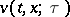,,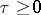,, for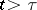, be a solution of the homogeneous equationand let it satisfy, for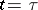, the initial conditions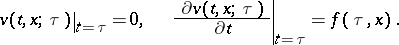Then the solution of the Cauchy problem (1), (2) is given by the Duhamel integral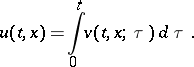This theorem, known as Duhamel's principle, is an analogue of the method of variation of constants.

A similar construction can be used for the Cauchy problem with a homogeneous initial condition for the equationwhereis a linear differential operator with coefficients independent of, containing derivatives with respect to the variableonly.

The solution of the Cauchy problem with homogeneous initial conditions for the inhomogeneous heat equation is expressed by the Duhamel integral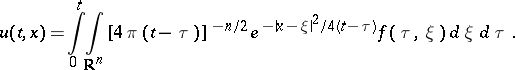For the wave equation ifone has: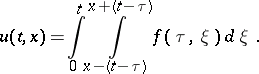The integral is named after J. Duhamel.

How to Cite This Entry:
Duhamel integral. Encyclopedia of Mathematics. URL: http://encyclopediaofmath.org/index.php?title=Duhamel_integral&oldid=12182
This article was adapted from an original article by A.K. Gushchin (originator), which appeared in Encyclopedia of Mathematics - ISBN 1402006098. See original article